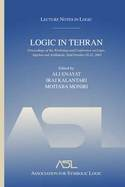# Lecture Notes in Logic, 26

## Logic in Tehran

Proceedings of the workshop and conference on logic, algebra, and arithmetic, held October 18-22, 2003

Ali Enayat, Iraj Kalantari, Mojtaba Moniri, editorsYear: 2006

ISBN: 1-56881-296-5
361 pages. Paperback.

ISBN:1-56881-295-7
361 pages. Hardcover.

This proceedings volume contains research papers in mathematical logic, especially in model theory and its applications to algebra and formal theories of arithmetic. Other papers address interpretability theory, computable analysis, modal logic, and the history of mathematical logic in Iran. The conference was held in Tehran, Iran, in October 2003, with the expressed purpose of bringing together researchers with connections to Iranian logicians and promoting further research in mathematical logic in Iran.

• Preface
• Workshop and conference on logic, algebra, and arithmetic:Tehran, October 18-22, 2003
Mathematical logic in Iran: A perspective
• Seyed Masih Ayat and Mojtaba Moniri
Real closed fields and IP-sensitivity
Categoricity and quantifier elimination for intuitionistic theories
• Darko Biljakovc, Mikhail Kochetov, and Salma Kuhlmann
Primes and irreducibles in truncation interger parts of real closed fields
• Lou van den Dries
On explicit definability in arithmetic
• Ali Enayat
From bounded arithmetic to second order arithmetic via auotmorphisms
• Yuri L. Ershov
Local-global principles and approximation theorems
• Mehdi Ghasemi and Mojtaba Moniri
Beatty sequences and the arithmetical hierarchy
• Iraj Kalantari and Larry Welch
Specker’s theorem, cluster points, and computable quantum functions
• Franz-Viktor Kuhlmann
Additive polynomials and their role in the model theory of valued fields
• Franz-Viktor Kuhlmann
Dense subfields of henselian fields, and integer parts
• Shahram Mohsenipour
A recursive nonstandard model for open induction with GCD property and cofinal primes
• Morteza Moniri
Model thoery of bounded arithmetic with applications to independence results
• Zia Movahed
Ibn-Sina’s anticipation of the formulas of Buridan and Barcan
• Anand Pillay
Remarks on algebraic D-varieties and the model theory of differential fields
• Massoud Pourmahdian and Frank Wagner
A simple positive Robinson theory with LSTP \$\not=\$ STP
• Albert Visser
Categories of theories and interpretations